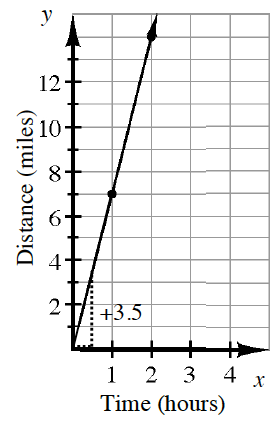### Home > ACC6 > Chapter 8 Unit 8 > Lesson CC1: 8.3.2 > Problem8-102

8-102.

The graph represents this situation: Cara jogs every morning, and after $30$ minutes, she has run $3.5$ miles.

1. How is Cara’s speed represented on the graph?

The steepness of the line represents how fast Cara is going.

2. Write a rule for Cara’s distance if $t$ represents the number of hours.

How far does Cara travel each hour? How does the distance traveled each hour relate to the total distance run and the time spent running?

d = 7t

3. If Cara continues to run at this rate, predict how far she will run in $4$ hours.

Use the answer from part (b) to help solve